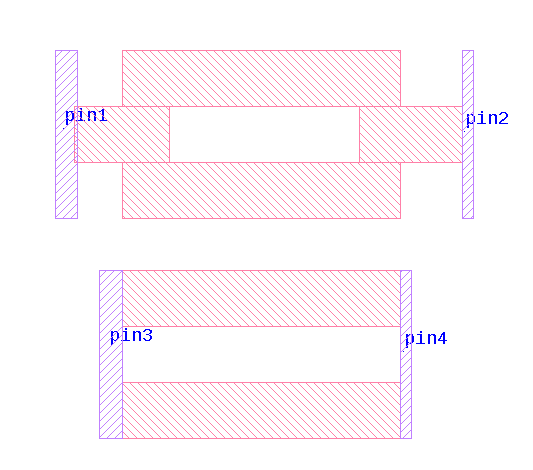# Resistor extractor - negative value

Hello Mattias,

I noted an issue with resistance value for resistor extractor. For very large resistor resistance have a negative sign in the netlist.
The absolute value is not correct.

I suspect that there might be a problem with memory or data type used in the extractor.

I printed some data in the console:
1) big resistor - negative value
circuit TOP ();
device RES \$1 (A=(null),B=(null)) (R=-50677.1781,L=-1013543.562,W=20,A=11340481.8183,P=2267920.18);
end;

2) smaller resistor - positive value - this works well
circuit TOP ();
device RES \$1 (A=(null),B=(null)) (R=47121.1641,L=942423.282,W=20,A=9425132.84231,P=1884886.561);
end;

I could send you the example design if you could tell me how.

Thank you,
Lidia

• Why is your L negative? That's the problem.

Now given that coords are 32-bit fixed in DBU, might you be using a too-small DBU and a too-long resistor body such that you "wrap around" to 2's complement negative sign, just by bits?

Now that shouldn't be let to happen but testing and trapping for what should be prevented by some combo of setup and common sense would probably burden performance quite a bit.

How about trying (say) a couple of things?
One, look at the polygon defining the resistor body. Look at the geom properties, the length and width in the second tab. Is -that- negative? Or is the negative value being developed within the extractor module?

Two, try making the resistor (say) 3 stripes at 1/3 the length, 9 at 1/9, ... And see if you can find a practical Lmax (for the DBU setup) and then change the DBU 10X and repeat for the 1/1 3/(1/3), 9/(1/9) cases to see if that's the handle. If so then you have to determine what a tenable DBU is, according to whoever is going do the physical manufacturing.
• It doesn't matter if there is a single wire between contacts or more wires only the total wires length between two contacts matters and is limited to:
Lmax = 1073741.5um (0.001um DBU)
Lmax = 10737418.0um (0.01um DBU)
I'm using 64bit Klayout version.

• edited August 2022

I can use dbu() command at the beginning of lvs script to move to bigger dbu only for lvs check and this should works for me.
Thanks !

• You really mean your resistor has a length of more than a meter?

I assume there is an integer overflow. I need to check this. 64bit KLayout is not the same than 64bit coordinate KLayout. 64bit coordinates enable a virtually unlimited coordinate space, but it comes with some problems in other places. So I'd not recommend that path.

The DBU value can (and should be) be increased if your layout is coarse (e.g. micrometer accuracy, not nm resolution). If that is sufficient you may want to increase your DBU in the layout to 10nm, 100nm or even larger. That will also allow larger layouts in the meter range.

Matthias

• edited August 2022

I wanted to use resistor extractor to extract the metal resistance, so 1m is not the resistor length, but routing length.
I found the problem in my approach as everything what is connected between resistor contacts will act as a serial connection.
I thought that resistance between pin1 and pin2 will be similar as between pin3 and pin4, but this is not true...This is why I have in the extractor length more than 1m. I need to change the lvs script to recognize all nodes as contacts...

Lidia

• edited September 2022

Hi Lida,

I see. That is actually a problem with the measurement function - it uses a length approximation which is only valid for single paths. Specifically, it computes the length and width from area and perimeter.

Your application is something that requires a decomposition of polygons and is more within the domain of parasitic extraction tools and not supported by the resistor extractor currently.

Anyway, thank you for this insight.

Matthias

• Practically all design (not parasitic) resistors (their "bodies", or, more specifically, seed layers/shapes) are simple rectangles - poly, diffusion, well, hi-res metal, LVS resistors, etc.

• Thank you both for the clarification.
Lidia